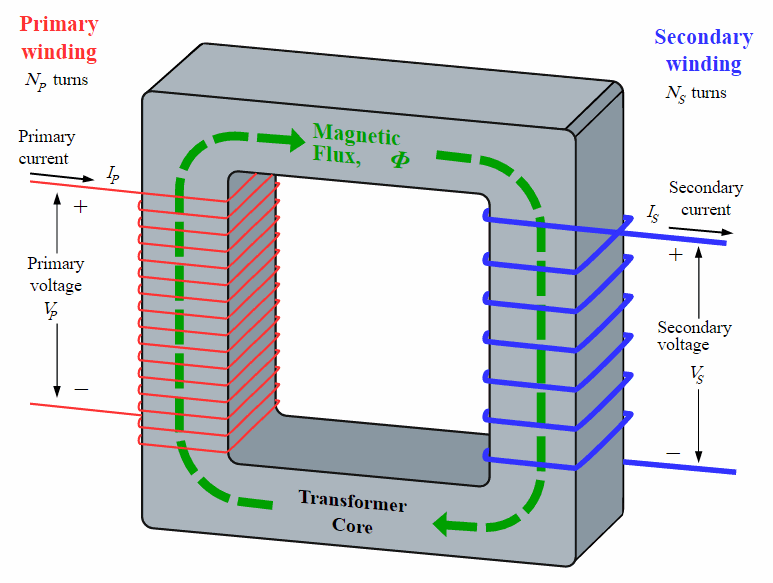Experiments with magnets and our surroundings

Twelve Fundamentals of Magnetism

11.   Transformer Action

http://en.wikipedia.org/wiki/Electromagnetic_induction

https://en.wikipedia.org/wiki/Transformer
b. What does this mean?

A voltage can be induced within a wire if it experiences a changing magnetic field.
This can be considered a Transformer Action since this is how transformers operate.

This phenomenon was discovered by Michael Faraday in 1831 and Joseph Henry in 1832, independently of each other.

d. How much voltage is induced?

The magnitude of the induced voltage, Ei = N*dΦ/dt

Where:
i. Ei is the induced voltage, Volts

ii. N is the number of turns of wire seeing the changing magnetic field

iii. d
Φ is the change in the magnetic field, Wb

iv. dt is the change in time, sec

A very simple equation!  The voltage induced is only affected by the the number of turns of wire, and how fast the magnetic field is changing.

e. What if the field is changing sinusoidally?

Since the voltage generated by the utility is sinusoidal in shape, how does this affect the above equation?

The rms value of the induced voltage, Erms = 4.44*f*N*
Φmax for a sinusoidally changing flux

Where:

i. Erms is the rms value of the induce voltage

ii. 4.44 is derived from 2*pi/sqrt(2)

iii. f is the frequency of the flux, Hz

iv. N is the number of turns of wire around an iron core

v.
Φmax is the maximum or peak value of the flux that is changing in a sinusoidal fashion, which is the same as Bmax*Ac

1. Bmax is the maximum or peak value of the flux density, Tesla
2. Ac is the area of the iron core, meter2Note that the current in the secondary circuit will create a magnetic flux that opposes the magnetic flux created by the current in the primary circuit.